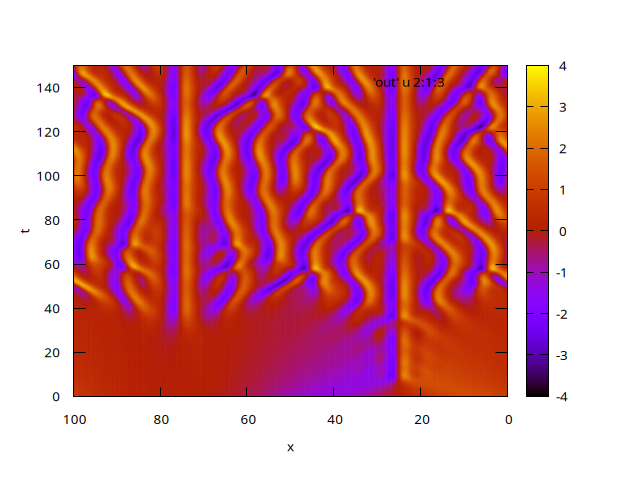# Kuramoto–Sivashinsky equation

Duchemin et Eggers, 2014, section 6 propose to use their “Explicit-Implicit-Null” method to solve the Kuramoto–Sivashinsky equation \displaystyle \partial_t u = -u \partial_x u - \partial^2_x u - \partial^4_x u while avoiding the stringent explicit timestep restriction due to the fourth derivative.

In this example we show that this can also be done using the implicit multigrid solver.

#include "grid/multigrid1D.h"
#include "solve.h"

This is the simple explicit discretisation (which is not used).

void solve_explicit (scalar u, double dt)
{
scalar du[];
foreach()
du[] = - u[]*(u - u[-1])/(2.*Delta)
- (u[-1] - 2.*u[] + u)/sq(Delta)
- (u[-2] - 4.*u[-1] + 6.*u[] - 4.*u + u)/sq(sq(Delta));
foreach()
u[] += dt*du[];
}

int main()
{

We reproduce the same test case as in section 6.2 of Duchemin & Eggers.

  init_grid (512);
L0 = 32.*pi;
periodic (right);
scalar u[];
foreach()
u[] = cos(x/16.)*(1. + sin(x/16.));

The timestep is set to 0.1, which is significantly larger than that in Duchemin & Eggers (0.014).

  double dt = 1e-1;
//  double dt = 1.4e-4;
int i = 0;
TOLERANCE = 1e-6;
for (double t = 0; t <= 150; t += dt, i++) {
if (i % 1 == 0) {
foreach()
fprintf (stdout, "%g %g %g\n", t, x, u[]);
fputs ("\n", stdout);
}
scalar b[];
foreach()
b[] = u[] - dt*u[]*(u - u[-1])/(2.*Delta);
solve (u,
u[] + dt*(u[-1] - 2.*u[] + u)/sq(Delta)
+ dt*(u[-2] - 4.*u[-1] + 6.*u[] - 4.*u + u)/sq(sq(Delta)),
b);
fprintf (stderr, "%g %d\n", t, solve_stats.i);
//    solve_explicit (u, dt);
}
}

The result can be compared to Figure 8 of Duchemin & Eggers.

set term PNG enhanced font ",10"
set output 'sol.png'
set pm3d map
set xlabel 'x'
set ylabel 't'
set xrange [100:0]
set yrange [0:150]
splot 'out' u 2:1:3Solution of the Kuramoto–Sivashinski equation (script)

 [duchemin2014] Laurent Duchemin and Jens Eggers. The explicit–implicit–null method: Removing the numerical instability of pdes. Journal of Computational Physics, 263:37–52, 2014. [ .pdf ]